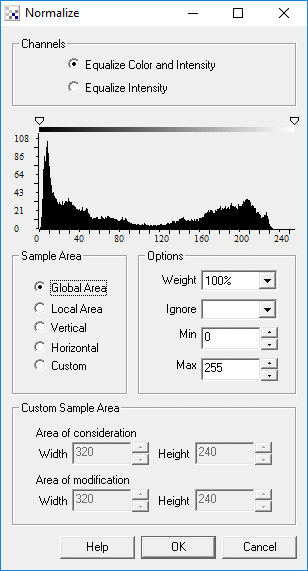# Normalize

The Normalize module stretches an image's pixel values to cover the entire pixel value range (0-255).

The function processes each color band (RGB) and determines the minimum and maximum value in each of the three color bands. Once these values are computed the image is reprocessed by subtracting the minimum value of each band from each pixel and dividing by its max-min range (3 times for each RGB pixel). This has the effect of stretching the pixel value to the full 0-255 pixel value range. Visually the image appears to have increased in contrast.

R=((R-Rmin)/(Rmax-Rmin))*255.0

G=((G-Gmin)/(Gmax-Gmin))*255.0

B=((B-Bmin)/(Bmax-Bmin))*255.0

Normalization is a good tool to combat lighting changes as the camera moves. Many filters rely on absolute pixel values (such as threshold) that are easily changed in different lighting conditions. Normalization is one of the tools used to reduce this sensitivity to lighting conditions.

## Interface## Instructions

1. Channel - Specify which channels (color and/or intensity) should be modified by the normalization module.

2. Sample Area - Specify which area is checked when performing the histogram equalization.

Global Area - consider the entire image when performing histogram normalization.
Local Area - consider a 20x20 area when performing histogram normalization. Note that this selection will execute VERY SLOWLY due to the per pixel computational requirements.
Vertical - consider the vertical column when performing histogram normalization.
Horizontal - consider the horizontal column when performing histogram normalization.
Custom - create your own block sizes. The 'Area of Consideration' specifies the window size of those pixels that will contribute to the normalization process. The 'Area of Modification' specifies the window of those pixels that will be modified based on the min/max values calculated from the 'Area of Consideration'. The two window sizes allow you to specify an overlapping processing which can help to smooth the individual results better.

3. Weight - specifies how much normalization the image should receive. At 100% the image is changed to the normalized values. At 20% the image pixels are changed by 20% towards the normalized values. The weighting is most useful when in the "Local Area" mode since a 100% normalization can produce undesired effects.

4. Ignore - specify the lower percent values of the histogram in which to ignore when determining the minimum and maximum levels to use in normalization. At 5% any pixels that are less than 5% in count relative to the maximum pixel count is ignored and not considered for the minimum and maximum range. This allows low pixel counts to be ignored during normalization. For example, this helps to prevent a single white pixel from forcing the maximum normalization value to be 255.

5. Min/Max - enforces limits on how wide the min and max range of the pixel values will be. For images that require normalization only in the high white pixels you can decrease the Max value which according to the above formula will limit the amount of normalization that can occur. The effect (similar to the Ignore setting) will reduce normalization noise in lower/darker pixels.

## Example

 Source Image Local Normalized Image at 80% weightIntensity Only Normalized Image